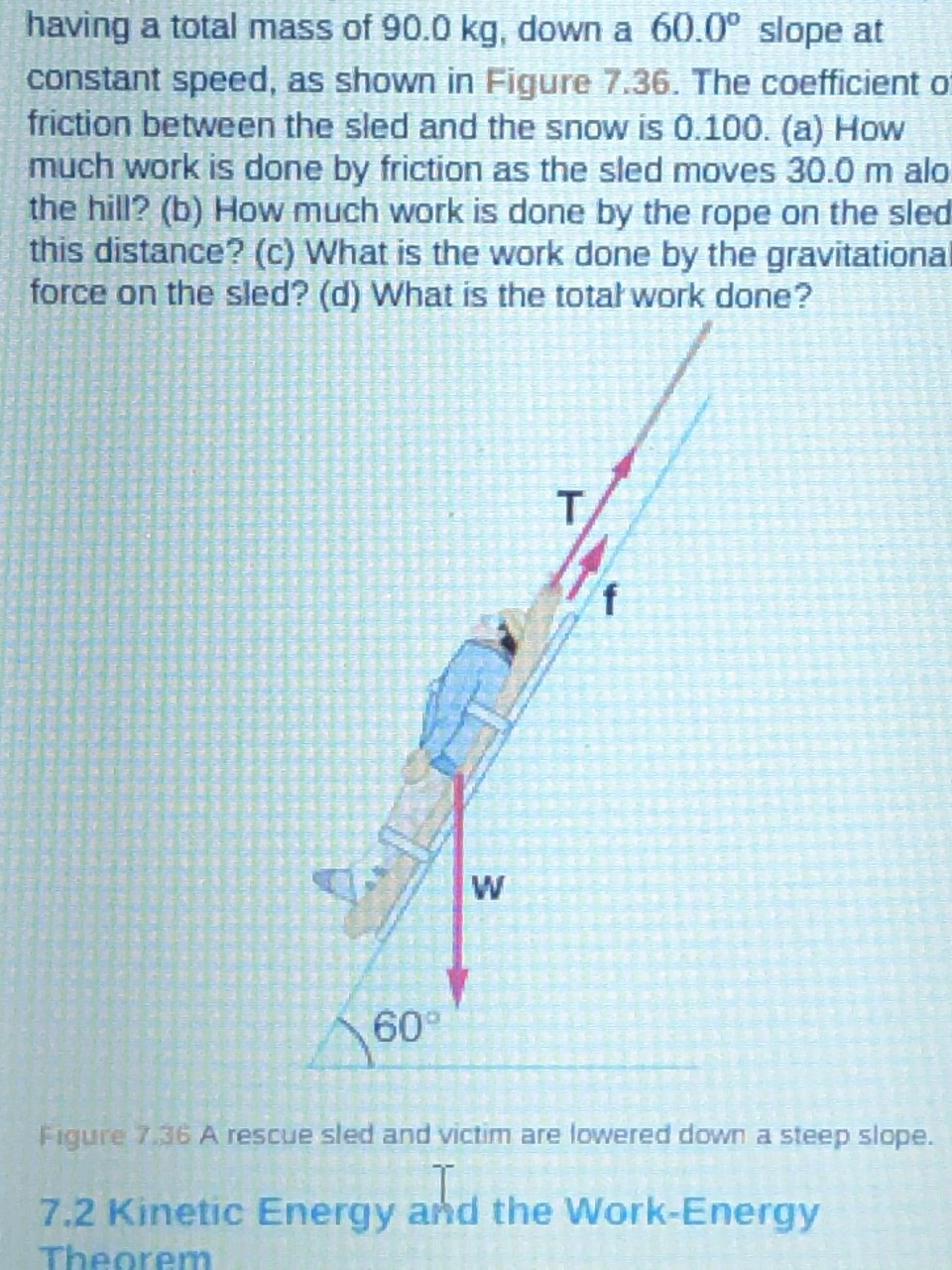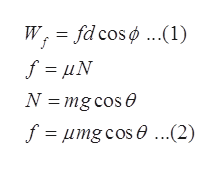# having a total mass of 90.0 kg, down a 60.0° slope atconstant speed, as shown in Figure 7.36. The coefficient offriction between the sled and the snow is 0.100. (a) Howmuch work is done by friction as the sled moves 30.0 m alo.the hill? (b) How much work is done by the rope on the sledthis distance? (c) What is the work done by the gravitationalforce on the sled? (d) What is the total work done?T,W60Figure 7.36 A rescue sled and victim are lowered down a steep slope.7.2 Kinetic Energy and the Work-EnergyTheorem

Question
1113 views

Suppose the ski patrol lowers a rescue sled and victim,
having a total mass of 90.0 kg, down a 60.0º slope at
constant speed, as shown in Figure 7.36. The coefficient of
friction between the sled and the snow is 0.100. (a) How
much work is done by friction as the sled moves 30.0 m along
the hill? (b) How much work is done by the rope on the sled in
this distance? (c) What is the work done by the gravitational
force on the sled? (d) What is the total work done?help_outlineImage Transcriptionclosehaving a total mass of 90.0 kg, down a 60.0° slope at constant speed, as shown in Figure 7.36. The coefficient of friction between the sled and the snow is 0.100. (a) How much work is done by friction as the sled moves 30.0 m alo. the hill? (b) How much work is done by the rope on the sled this distance? (c) What is the work done by the gravitational force on the sled? (d) What is the total work done? T, W 60 Figure 7.36 A rescue sled and victim are lowered down a steep slope. 7.2 Kinetic Energy and the Work-Energy Theorem fullscreen
check_circle

Step 1

Since we only answer up to 3 sub-parts, we’ll answer the first 3. Please resubmit the question and specify the other subparts (up to 3) you’d like answered.

Here, the θ is the angle of the slope with horizontal, f is the frictional force, T is the tension, N is normal reaction, mg is the weight. The free body diagram for the given sled problem is:

Step 2

Given,

Step 3

The work done by frictional force (Wf) is the dot product of the frictional force (f) and the displacement (d), where φ is t...help_outlineImage Transcriptionclosefd cos ø..1) W, f= μN N mg cos f = umg cos e ...(2) e fullscreen

### Want to see the full answer?

See Solution

#### Want to see this answer and more?

Solutions are written by subject experts who are available 24/7. Questions are typically answered within 1 hour.*

See Solution
*Response times may vary by subject and question.
Tagged in

### Physics